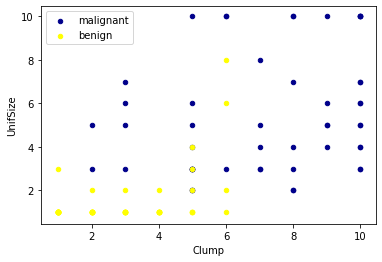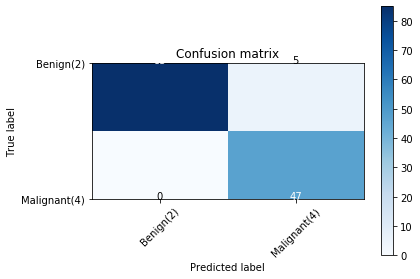0%

In this notebook, you will use SVM (Support Vector Machines) to build and train a model using human cell records, and classify cells to whether the samples are benign or malignant.

SVM works by mapping data to a high-dimensional feature space so that data points can be categorized, even when the data are not otherwise linearly separable. A separator between the categories is found, then the data is transformed in such a way that the separator could be drawn as a hyperplane. Following this, characteristics of new data can be used to predict the group to which a new record should belong.

In :
import pandas as pd
import pylab as pl
import numpy as np
import scipy.optimize as opt
from sklearn import preprocessing
from sklearn.model_selection import train_test_split
%matplotlib inline
import matplotlib.pyplot as plt


The example is based on a dataset that is publicly available from the UCI Machine Learning Repository (Asuncion and Newman, 2007)[http://mlearn.ics.uci.edu/MLRepository.html]. The dataset consists of several hundred human cell sample records, each of which contains the values of a set of cell characteristics. The fields in each record are:

Field name Description
ID Clump thickness
Clump Clump thickness
UnifSize Uniformity of cell size
UnifShape Uniformity of cell shape
SingEpiSize Single epithelial cell size
BareNuc Bare nuclei
BlandChrom Bland chromatin
NormNucl Normal nucleoli
Mit Mitoses
Class Benign or malignant

For the purposes of this example, we're using a dataset that has a relatively small number of predictors in each record. To download the data, we will use !wget to download it from IBM Object Storage.
Did you know? When it comes to Machine Learning, you will likely be working with large datasets. As a business, where can you host your data? IBM is offering a unique opportunity for businesses, with 10 Tb of IBM Cloud Object Storage: Sign up now for free

In :
#Click here and press Shift+Enter
!wget -O cell_samples.csv https://s3-api.us-geo.objectstorage.softlayer.net/cf-courses-data/CognitiveClass/ML0101ENv3/labs/cell_samples.csv

--2020-05-08 02:29:54--  https://s3-api.us-geo.objectstorage.softlayer.net/cf-courses-data/CognitiveClass/ML0101ENv3/labs/cell_samples.csv
Resolving s3-api.us-geo.objectstorage.softlayer.net (s3-api.us-geo.objectstorage.softlayer.net)... 67.228.254.196
Connecting to s3-api.us-geo.objectstorage.softlayer.net (s3-api.us-geo.objectstorage.softlayer.net)|67.228.254.196|:443... connected.
HTTP request sent, awaiting response... 200 OK
Length: 20675 (20K) [text/csv]
Saving to: ‘cell_samples.csv’

cell_samples.csv    100%[===================>]  20.19K  --.-KB/s    in 0.02s

2020-05-08 02:29:54 (1003 KB/s) - ‘cell_samples.csv’ saved [20675/20675]



### Load Data From CSV File

In :
cell_df = pd.read_csv("cell_samples.csv")

Out:
ID Clump UnifSize UnifShape MargAdh SingEpiSize BareNuc BlandChrom NormNucl Mit Class
0 1000025 5 1 1 1 2 1 3 1 1 2
1 1002945 5 4 4 5 7 10 3 2 1 2
2 1015425 3 1 1 1 2 2 3 1 1 2
3 1016277 6 8 8 1 3 4 3 7 1 2
4 1017023 4 1 1 3 2 1 3 1 1 2

The ID field contains the patient identifiers. The characteristics of the cell samples from each patient are contained in fields Clump to Mit. The values are graded from 1 to 10, with 1 being the closest to benign.

The Class field contains the diagnosis, as confirmed by separate medical procedures, as to whether the samples are benign (value = 2) or malignant (value = 4).

Lets look at the distribution of the classes based on Clump thickness and Uniformity of cell size:

In :
ax = cell_df[cell_df['Class'] == 4][0:50].plot(kind='scatter', x='Clump', y='UnifSize', color='DarkBlue', label='malignant');
cell_df[cell_df['Class'] == 2][0:50].plot(kind='scatter', x='Clump', y='UnifSize', color='Yellow', label='benign', ax=ax);
plt.show()## Data pre-processing and selection

Lets first look at columns data types:

In :
cell_df.dtypes

Out:
ID              int64
Clump           int64
UnifSize        int64
UnifShape       int64
SingEpiSize     int64
BareNuc        object
BlandChrom      int64
NormNucl        int64
Mit             int64
Class           int64
dtype: object

It looks like the BareNuc column includes some values that are not numerical. We can drop those rows:

In :
cell_df = cell_df[pd.to_numeric(cell_df['BareNuc'], errors='coerce').notnull()]
cell_df['BareNuc'] = cell_df['BareNuc'].astype('int')
cell_df.dtypes

Out:
ID             int64
Clump          int64
UnifSize       int64
UnifShape      int64
SingEpiSize    int64
BareNuc        int64
BlandChrom     int64
NormNucl       int64
Mit            int64
Class          int64
dtype: object
In :
feature_df = cell_df[['Clump', 'UnifSize', 'UnifShape', 'MargAdh', 'SingEpiSize', 'BareNuc', 'BlandChrom', 'NormNucl', 'Mit']]
X = np.asarray(feature_df)
X[0:5]

Out:
array([[ 5,  1,  1,  1,  2,  1,  3,  1,  1],
[ 5,  4,  4,  5,  7, 10,  3,  2,  1],
[ 3,  1,  1,  1,  2,  2,  3,  1,  1],
[ 6,  8,  8,  1,  3,  4,  3,  7,  1],
[ 4,  1,  1,  3,  2,  1,  3,  1,  1]])

We want the model to predict the value of Class (that is, benign (=2) or malignant (=4)). As this field can have one of only two possible values, we need to change its measurement level to reflect this.

In :
cell_df['Class'] = cell_df['Class'].astype('int')
y = np.asarray(cell_df['Class'])
y [0:5]

Out:
array([2, 2, 2, 2, 2])

## Train/Test dataset

Okay, we split our dataset into train and test set:

In :
X_train, X_test, y_train, y_test = train_test_split( X, y, test_size=0.2, random_state=4)
print ('Train set:', X_train.shape,  y_train.shape)
print ('Test set:', X_test.shape,  y_test.shape)

Train set: (546, 9) (546,)
Test set: (137, 9) (137,)


## Modeling SVM

The SVM algorithm offers a choice of kernel functions for performing its processing. Basically, mapping data into a higher dimensional space is called kernelling. The mathematical function used for the transformation is known as the kernel function, and can be of different types, such as:

1.Linear
2.Polynomial
4.Sigmoid


Each of these functions has its characteristics, its pros and cons, and its equation, but as there's no easy way of knowing which function performs best with any given dataset, we usually choose different functions in turn and compare the results. Let's just use the default, RBF (Radial Basis Function) for this lab.

In :
from sklearn import svm
clf = svm.SVC(kernel='rbf')
clf.fit(X_train, y_train)

/home/jupyterlab/conda/envs/python/lib/python3.6/site-packages/sklearn/svm/base.py:196: FutureWarning: The default value of gamma will change from 'auto' to 'scale' in version 0.22 to account better for unscaled features. Set gamma explicitly to 'auto' or 'scale' to avoid this warning.
"avoid this warning.", FutureWarning)

Out:
SVC(C=1.0, cache_size=200, class_weight=None, coef0=0.0,
decision_function_shape='ovr', degree=3, gamma='auto_deprecated',
kernel='rbf', max_iter=-1, probability=False, random_state=None,
shrinking=True, tol=0.001, verbose=False)

After being fitted, the model can then be used to predict new values:

In :
yhat = clf.predict(X_test)
yhat [0:5]

Out:
array([2, 4, 2, 4, 2])

## Evaluation

In :
from sklearn.metrics import classification_report, confusion_matrix
import itertools

In :
def plot_confusion_matrix(cm, classes,
normalize=False,
title='Confusion matrix',
cmap=plt.cm.Blues):
"""
This function prints and plots the confusion matrix.
Normalization can be applied by setting normalize=True.
"""
if normalize:
cm = cm.astype('float') / cm.sum(axis=1)[:, np.newaxis]
print("Normalized confusion matrix")
else:
print('Confusion matrix, without normalization')

print(cm)

plt.imshow(cm, interpolation='nearest', cmap=cmap)
plt.title(title)
plt.colorbar()
tick_marks = np.arange(len(classes))
plt.xticks(tick_marks, classes, rotation=45)
plt.yticks(tick_marks, classes)

fmt = '.2f' if normalize else 'd'
thresh = cm.max() / 2.
for i, j in itertools.product(range(cm.shape), range(cm.shape)):
plt.text(j, i, format(cm[i, j], fmt),
horizontalalignment="center",
color="white" if cm[i, j] > thresh else "black")

plt.tight_layout()
plt.ylabel('True label')
plt.xlabel('Predicted label')

In :
# Compute confusion matrix
cnf_matrix = confusion_matrix(y_test, yhat, labels=[2,4])
np.set_printoptions(precision=2)

print (classification_report(y_test, yhat))

# Plot non-normalized confusion matrix
plt.figure()
plot_confusion_matrix(cnf_matrix, classes=['Benign(2)','Malignant(4)'],normalize= False,  title='Confusion matrix')

              precision    recall  f1-score   support

2       1.00      0.94      0.97        90
4       0.90      1.00      0.95        47

micro avg       0.96      0.96      0.96       137
macro avg       0.95      0.97      0.96       137
weighted avg       0.97      0.96      0.96       137

Confusion matrix, without normalization
[[85  5]
[ 0 47]]You can also easily use the f1_score from sklearn library:

In :
from sklearn.metrics import f1_score
f1_score(y_test, yhat, average='weighted')

Out:
0.9639038982104676

Lets try jaccard index for accuracy:

In :
from sklearn.metrics import jaccard_similarity_score
jaccard_similarity_score(y_test, yhat)

Out:
0.9635036496350365

## Practice

Can you rebuild the model, but this time with a linear kernel? You can use kernel='linear' option, when you define the svm. How the accuracy changes with the new kernel function?

In :
# write your code here